# Basic Equations for Parker Pneumatic Rotary Actuator

### Mass Moments of Inertia Equations Table

 Rectangular Prism Ix = 1/12m(b2 + c2) Iy = 1/12m(c2 + a2) Iz = 1/12m(a2 + b2 )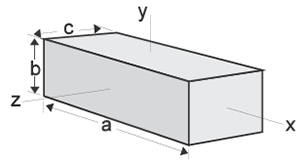Circular Cylinder Ix = 1/2ma2 Iy = Iz = 1/2m(3a2 + L2)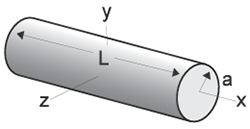Thin Rectangular Plate Ix = 1/12m(b2 + c2) Iy = 1/12mc2 Iz = 1/12mb2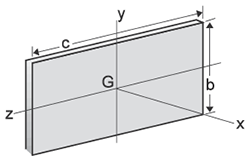Circular Cone Ix = 3/10ma2 Iy = Iz = 3/5m(1/4a2 + h2)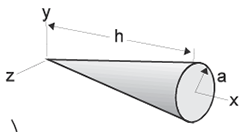Sphere Ix = Iy = Iz = 2/5ma2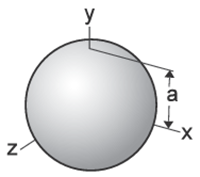Thin Disk Ix = 1/2mr2 Iy = Iz = 1/4mr2Parallel Axis Theorem Ip = ⌈ + md2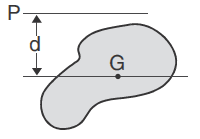Ip = Mass moment of inertia about an axis parallel to a centroidal axis ⌈ = Mass moment of inertia about a centroidal axis m = Mass d = Distance between axes

Where:

t = time

θ = angular position

ωt = angular velocity at time = t

ω0 = angular velocity at time = 0

α = angular acceleration

### When Acceleration Is Constant:

θ = ω0t + 1/2αt2 ;   α = 2θ/t2

θ = ω0t + 1/2ωtt ;  α = (ωt - ω0)2/2θ

ω = (ω02 + 2αθ)1/2 ; α = (ωt - ω0)/t

θ = ωt

### Basic Velocity, Acceleration, Kinetic Energy and Torque Equations

(The equations below are based on triangular velocity profile.)

Where:

Θ = Angle of rotation (degrees)

t = Time to rotate through Θ (sec)

WL = Weight of load (lbf)

Ta = Torque to accelerate load (lb-in)

Us = Coefficient of static friction

Jm* = Rotational mass moment of inertia (lb-in-sec2)

Tf = Torque to overcome friction (lb-in)

TL = Torque to overcome effects of gravity

* Use "I" values from the Mass Moments of Inertia table

Equations:

ωmax = 0.35 x Θ/t

α = ωmax2/ (Θ/57.3)

α = ωmax/(t/2)

K.E. = 1/2 Jmω2

Ta = α x Jm

Tf = W x Us x (Distance from pivot point to center of external bearings)

TL = (Torque arm length to C.G. of load) x WL x cos (Φ)

(Where Φ = Angle between torque arm and horizontal plane)

### Coefficients of Friction

 Material* μs μk Steel on steel 0.80 0.40 Steel on steel (lubricated) 0.16 0.03 Aluminum on steel 0.45 0.30 Copper on steel 0.22 0.22 Brass on steel 0.35 0.19 PTFE on steel 0.04 0.04

*Dry contact unless noted

## Available Pneumatic Cylinder Styles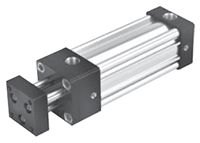Tie Rod Cylinders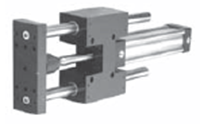Guided Cylinders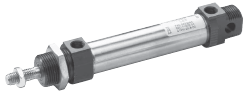Round Body Cylinders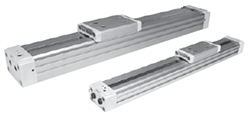Rodless Cylinders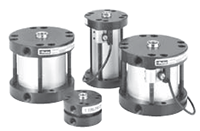Compact Cylinders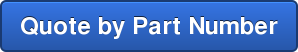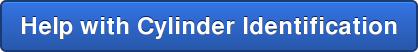## Engineering & Product Selection Information

### Pneumatic Product Selection

• Pneumatic Actuators & Air Cylinders
• Pneumatic Cylinders
• Automation Products: rotary actuators, grippers, slide tables, rotary tables, escapement
• Actuator Accessories: Linear alignment couplers, flow controls, air oil tanks, rodlocks, electronic sensors, shock absorbers

### Application Engineering Data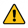WARNING: These products can expose you to chemicals including lead and/or DEHP and/or carbon black, which are known to the State of California to cause cancer or birth defects or other reproductive harm.  For more information go to www.P65Warnings.ca.gov